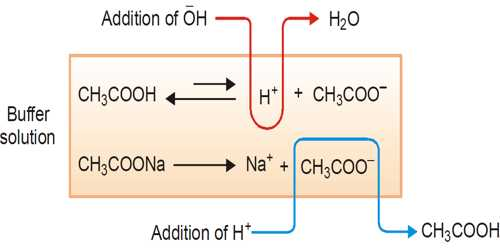# Examples of Buffer Action

Examples of buffer action

The ability of the buffer solution to resist the changes in pH value on the addition of small amount of an acid or a base is known as buffer action.

A buffer solution is one which resists changes in pH when small quantities of an acid or an alkali are added to it. An acidic buffer solution is simply one which has a pH less than 7. Acidic buffer solutions are commonly made from a weak acid and one of its salts – often a sodium salt.

The effect of the addition of a small amount of acid or alkali on the pH (= 4.93) of the buffer solution mentioned in example illustrates its buffering action.

(a) Let us calculate the change in pH of this buffer when 1.0 ml of 1.0 mol L-1 HCl solution is added to 1.0 L of buffer. The number of moles of HCl added is-

(1.0 x 1.0) / 1000 = 0.001

This amount of acid will combine with CH3COO ion to form CH3COOH. Hence the concentration of CH3COOH increases by 0.001 to 0.101 mol L-1 and the concentration of CH3COO, i.e. the concentration of salt decreases by 0,001 mol to (0.15 — 0.001 =) 0.149 mol L-1. Using these values in the equation we get,

pH = pKa + log (0.149/0.010)

= 4.75 + log 1.475

= 4.75+ log 1.475

= 4.75 + 0.168 = 4.92

(b) Now let us calculate the change in the pH of this buffer when 1.0 ml. of 1.0 mol L-1 of NaOH solution is added to this buffer. The number of moles of NaOH added is

(1.0 x 1.0) / 1000 = 0.001

This amount will react with CH3COOH to form water and CH3COO. Hence the concentration of CH3COOH will decrease by 0.001 to 0.099 mol L-1 and the concentration of CH3COO, i.e. the concentration of salt increases by 0.001 mol to (0.15 + 0.001 =) 0.151 mol L-1. Using these values in the equation (16.46) we get,

pH = pKa + log (0.151/0.099)

= 4.75 + log 1.525

= 4.75 + 0.18 = 4.93

So, we see that the addition of 0.001 mol of HCl or 0.001 mol NaOH to 1.0 L of the buffer has insignificant effect on its pH.